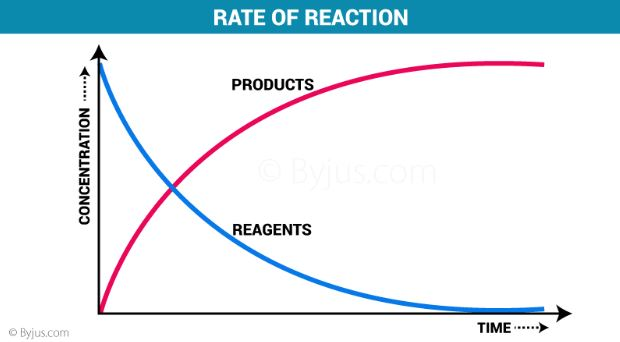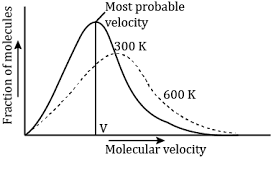Jet Set Go! All about Aeroplanes Jet Set Go! All about Aeroplanes

# Rate of Reaction Questions

The rate of a chemical reaction is defined as the ratio of the increase in product concentration per unit time to the decrease in reactant concentration per unit time. The rate of reaction varies greatly. For example, oxidative corrosion of iron in the Earth’s atmosphere takes many years, but cellulose combustion in a fire takes fractions of a second.Chemical kinetics is the branch of physical chemistry concerned with the measurement and prediction of chemical reaction rates, as well as the use of reaction-rate data to determine likely reaction processes. Chemical kinetics concepts are used in a variety of fields, including chemical engineering, enzymology, and environmental engineering.

 Definition: The rate of reaction, also known as reaction rate, is the rate at which reactants become products. When it comes to chemical reactions, it’s a given that the rate at which they happen varies significantly. Some chemical reactions occur almost instantly, while others take time to achieve their final equilibrium.

## Rate of Reaction Chemistry Questions with Solutions

Q1: Catalyst is a substance, which __________ chemical reaction.

a) Increases the speed of a

b) Decreases the speed of a

c) Can either increase or decrease the speed of a

d) Alters the value of equilibrium constant in a reversible

Answer: a) Increases the speed of a

Q2: A chemical reaction is of zero order, when the reaction rate is (where, CA = concentration of reactant)

a) ∝ CA

b) ∝ 1/CA

c) Independent of temperature

d) None of these

Q3: The rate law for the reaction involved in inversion of cane sugar is R = k [C12H22O11] [H2O].

a) True

b) False

Explanation: The reaction for the inversion of cane sugar would be C12H22O11 + H2O → glucose + fructose.

Even though the molecularity is 2, the reaction order is 1, hence the rate law R = k[C12H22O11] applies.

Q4: What is the rate law for acid hydrolysis of an ester such as CH3COOC2H5 in aqueous solution?

a) k [CH3COOC2H5]

b) k [CH3COOC2H5] [H2O]

c) k [CH3COOC2H5]2

d) k

Explanation: Acid hydrolysis of ester, CH3COOC2H5 + H2O → CH3COOH + C2H5OH

When one reactant is taken in excess of the other, the reaction’s order can be altered.

The rate law R= k [CH3COOC2H5] [H2O] even so water is present in excess.

As a result, R= k [CH3COOC2H5].

Q5: The rate constant of a reaction is k=3.28×10-4 s-1. Find the order of the reaction.

a) Zero order

b) First order

c) Second order

d) Third order

Explanation: Given, k= 3.28×10-4 s-1

The standard formula for calculating rate constant units is k=(mol L-1)1-ns-1, where ‘n’ is the reaction order. The value of ‘n’ must be 1 for (mol L-1)1-ns-1 to be s-1. As a result, k=3.28×10-4s-1 denotes a first-order reaction.

Q6: What are the units of a rate constant of a

1. First order reaction
2. nth order reaction.

1. First order reaction –

Units of rate constant, k = sec-1

1. For nth order reaction

the units of rate constant, k = mol (1-n) (n-1) Ls-1

Q7: What is the rate of chemical reaction?

The rate of reaction is defined as the change in reactant or product concentration per unit time. Any quantifiable physical property, such as colour, smell, or condition, can be used to measure changes in concentration.

Q8: Match the statements given in Column I and Column II

Column I

Column II

(a) Catalyst alters the rate of reaction

(i) cannot be fraction or zero

(b) Molecularity

(ii) proper orientation is not there always

(c) Second half-life of first order reaction

(iii) By lowering the activation energy

(d) e-Ea/RT

(iv) is same as the first

(e) Energetically favourable reactions are sometimes slow

(v) total probability is one

(f) Area under the Maxwell Boltzman curve is constant

(vi) refers to the fraction of molecules with energy equal to or greater than activation energy

(a) Catalyst alters the rate of reaction – (iii) By lowering the activation energy

(b) Molecularity – (i) cannot be fraction or zero

(c) Second half-life of first order reaction – (iv) is same as the first

(d) e-Ea/RT – (vi) refers to the fraction of molecules with energy equal to or greater than activation energy

(e) Energetically favourable reactions are sometimes slow – (ii) proper orientation is not there always

(f) Area under the Maxwell Boltzman curve is constant – (v) total probability is one

Q9: What do you understand by the rate law and rate constant of a reaction?

Identify the order of a reaction if the units of its rate constant are : (i) L-1 mol s-1 (ii) L mol-1 s-1

The rate of reaction is shown to be dependent on the concentration terms of reactant A and reactant B.

Then, Rate of reaction ∝ [A]α [B]β

or Rate = K [A]α [B]β

This expression is termed Rate law.

In this equation, ‘K’ stands for Rate constant. Units of rate constant are:

(i) Unit = L-1 mol s-1 → Zero order reaction

(ii) Unit = L mol-1 s-1 → Second order reaction.

Q10: Differentiate between Order and Molecularity of a reaction.

ORDER OF A REACTION

MOLECULARITY OF A REACTION

It is the sum of the concentration terms on which the rate of reaction is based, or the sum of the concentration exponents in the rate law equation.

It is the number of atoms, ions, or molecules that must collide at the same time in order for a chemical reaction to occur.

It does not have to be a whole number; it might be fractional or zero.

It is always a whole number.

It can only be determined through experiment and cannot be calculated.

It is simple to calculate by adding the molecules from the slowest step.

It is for the overall reaction and no individual steps are written to get it.

A complex reaction’s overall molecularity is irrelevant. Only the molecularity of the slowest step has any bearing on the entire process.

As the unbalanced equation shows, even the order of a simple reaction may not be equal to the number of molecules of the reactants.

The molecularity of simple reactions is usually determined by the equation’s Stoichiometry.

Q11: Derive an expression to calculate the time required for completion of a zero order reaction.

From the zero order reaction we know [A] = [A]0 – k t

For the completion, [A] = 0, therefore t = [A]0 / k

Q12: Define each of the following:

i) Specific rate of a reaction

ii) Activation energy of a reaction

i) Specific rate of a reaction

The rate of reaction is called the specific rate of reaction when the molar concentration of each of the reactants is equal.

ii) Activation energy of a reaction

The activation energy is the minimum additional amount of energy that the reactant molecules must absorb in order to reach the threshold value. The activation energy of the reaction is decreased when a catalyst is added.

Q13: How does collision theory explain the formation of products in a chemical reaction?

In Collision Theory, the reactant molecules are hard spheres, and the reaction occurs when they collide. Collisions between molecules with sufficient kinetic energy (called threshold energy) and suitable orientation cause the formation of a product. The activation energy and appropriate orientation of the molecules determine the conditions for effective collision and thus, the pace of a process.

Q14: What happens to the most probable kinetic energy and the energy of activation with increase in temperature?

The average kinetic energy of the reactive species increases as the temperature rises. The most probable kinetic energy is the peak of the curve, which corresponds to the kinetic energy of the maximum proportion of the molecule. The maximum of the curve increases towards higher energy as the temperature increases. At higher temperatures, the percentage of molecules with the most probable kinetic energy decreases.Q15: With the help of an example explain what is meant by pseudo first order reaction.

Consider the hydrolysis of ethyl acetate, which can be written as

CH3COOC2H5 + H2O → CH3COOH + C2H5OH

The rate equation is given as Rate = k [CH3COOC2H5] [H2O]

The concentration of water in this situation is relatively high, thus it does not change much during the procedure. As a result, the reaction rate is unaffected by changes in H2O concentration. In the following reaction, we might construct the effective rate equation as if the term representing a change in water concentration is set to zero.

Rate = k [CH3COOC2H5]

The term here refers to the value of the constant concentration of water.

where K = K’ [H2O]

As we can see, the reaction is a first-order reaction. These are known as pseudo-first-order reactions.

## Practise Questions on Rate of Reaction

Q1: What is the rate law for the reaction C2H4 + I2 → C2H4I2?

a) R= [C2H4] [I2]3/2

b) R= [C2H4] [I2]3

c) R= [C2H4] [I2]2

d) R= [C2H4] [I2]

Q2: The rate of a chemical reaction tells us about

a) the reactants taking part in the reaction

b) how slow or fast the reaction is taking place

c) the products formed in the reaction

d) none of the above

Q3: Define ‘activation energy’ of a reaction.

Q4: Write the rate equation for the reaction 2A + B → C if the order of the reaction is zero.

Q5: What is meant by Half-Life?

Click the PDF to check the answers for Practice Questions.

## Recommended Videos

#### Zero Order Reaction | CHEMISTRY |#### Initial Rate Method | CHEMISTRY |#### Chemical Kinetics and Surface Chemistry